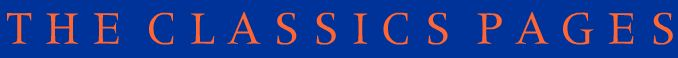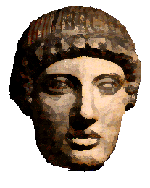# The Maths-Classics project

## Assignment 5

### The Greeks

There were many famous Greek Mathematicians. Write briefly about the following men: the information in brackets may help you.

PYTHAGORAS     ( theorem )

EUCLID     ( measuring the width of a river )

ARCHIMEDES     ( heureka ( which is the proper Greek word, rather than eureka!), principle, screw )

ERATOSTHENES ( circumference of the earth, sieve )

Draw each of the following . Find out the literal meaning and explain how the mathematical meaning has developed.

1. trapezium ( literally means 'table shape', why maths meaning? )2. rhombus

3. isosceles triangle

4. parallel lines

5. parallelogram

6. sphere

7. pyramid

8. tetrahedron

9. cone

10. cylinder

11. prism